# GED Math Practice Test 4:No Calculator

These free GED Math practice questions are designed to be solved without using a calculator. On the GED Math test the first 5 problems must be solved without the help of a calculator. These problems often involve number lines, fractions, common factors, multiplication, division, and undefined expressions. Our practice test includes a variety of challenging problems that you can use for your GED Math test prep.

Directions: Solve each problem without using a calculator.

Congratulations - you have completed . You scored %%SCORE%% out of %%TOTAL%%. Your performance has been rated as %%RATING%%
 Question 1
Two points are plotted on a number line: −11.2 and 3.3. What is the distance between these two points?

 A 7.9 B −7.9 C 14.5 D −14.5
Question 1 Explanation:
The distance between points A and B on a number line is the absolute value of the difference between those two points. Distance is always a positive value. Evaluate the difference between the points and then apply the absolute value:

AB = |ba| or |ab|

= |−11.2 − 3.3|
= |−14.5|
= 14.5

Recall that the absolute value of a number is its distance from 0.
 Question 2
15.05 ÷ 5 =

 A 3.1 B 0.301 C 3.01 D 0.501
Question 2 Explanation:
Rewrite the problem in long division form, ensuring that the decimal point in your answer is placed directly above the decimal point in the dividend (15.05):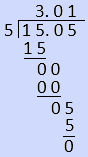Question 3
Which of the following expressions is undefined in the set of real numbers?

 ABCD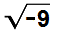Question 3 Explanation:
The square root of a number is the value that when multiplied with itself gives the number inside the square root, for example: √16 = ±4, because 4 * 4 = 16, and −4 * −4 = 16. Notice that only one answer choice shows a negative value inside the square root. This answer, √−9, must be correct, because there is no real number that when multiplied with itself will give −9.

A negative integer squared is positive and a positive integer squared is positive, so the square root of a negative value is undefined in the set of real numbers.
 Question 4
Find the greatest common factor of 33 and 44.

 A 1 B 11 C 22 D 33
Question 4 Explanation:
The factors of a number are values that divide evenly into that number. For example, the factors of 4 are 1, 2, and 4 because 4 can be divided by each without producing a remainder. To determine the greatest common factor of 33 and 44, begin by listing the factors of each number:

Factors of 33 are: 1, 3, 11, 33
Factors of 44 are: 1, 2, 4, 11, 22, 44

The greatest common factor is the largest number that is in both lists of factors: 11.
 Question 5
77 × 1.7 =

 A 177 B 61.6 C 139 D 130.9
Question 5 Explanation:
When multiplying decimal values together, right align the values vertically and multiply as usual, ignoring the decimal points until the product is found: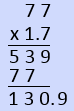Placement of the decimal point in the product is determined by the number of digits to the right of the decimal points in the values multiplied. In this case, there is one digit to the right of the decimal points: 7, so the product must also have one digit to the right of the decimal: 1309 becomes 130.9 to satisfy this condition.
 Question 6
What is the distance between the two points shown on the number line?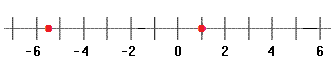A 5½ B 6½ C −5½ D 6
Question 6 Explanation:
Begin by labeling the point values. The number line shows markers every 1 space with labels every 2 spaces. The point halfway between −5 and −6 must be −5½, and the point midway between 0 and 2 must be 1. To determine the distance between these points, evaluate the difference and take the absolute value:

AB = |ba| or |ab|

= |−5½ − 1|
= |−6½|
= 6½

(Recall that subtracting a positive number is the same as adding its negative.)
 Question 7A Undefined B −2 C 2 D 4
Question 7 Explanation:
This problem is asking for the cube root of −8. The cube root of a number is the value that, when cubed (multiplied with itself 3 times), gives the number inside of the radical. Remember that a negative number raised to an even exponent gives a positive result, but when raised to an odd exponent gives a negative result. Consequently, the cube root of −8 will be a negative number. It should also be apparent that squaring 3 gives a value greater than 8, so cubing 3 would give a value much larger than 8. Trying −2 as the likely possibility:

−2 * −2 = 4 * −2 = −8. So, −2 is the cube root of −8.
 Question 8
Find the value for x that makes this fraction undefined.A 5 B 0 C −12 D −5
Question 8 Explanation:
A fraction is undefined for any value that where the denominator is equal to 0. To solve this problem, set the denominator equal to 0 and solve for the variable x:

5 + x = 0
x = −5
 Question 9
13.02 ÷ 2.1 =

 A 6.2 B 0.16 C 0.62 D 5.7
Question 9 Explanation:
This problem can be solved using long division. In order to perform long division when the divisor is a decimal value as it is here, it is necessary to first convert the divisor to a whole number. In this case, we can convert 2.1 to a whole number by multiplying it by 10; however, to ensure that we are not changing the problem, it is also necessary to multiply the dividend, 13.02, by 10. Rewriting the problem after this multiplication by 10: 130.2 ÷ 21.

From here, rewrite the problem into long division form, being sure to place the decimal in the answer directly above its place in the dividend, and dividing as usual:Question 10
Find the greatest common factor of 16, 27, and 20.

 A 1 B 4 C 8 D 9
Question 10 Explanation:
To evaluate the greatest common factor of the three numbers, begin by listing the factors of each number:

Factors of 16: 1, 2, 4, 8, 16
Factors of 20: 1, 2, 4, 5, 10, 20
Factors of 27: 1, 3, 9, 27

The greatest common factor is the largest number that goes into EACH of the original values. It can be seen that 1 is the only number that evenly divides into each number, as a result, 1 is the answer.
 Question 11
What is the value of this numerical expression?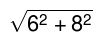A 7 B 14 C 10 D 100
Question 11 Explanation:
According to the order of operations (PEMDAS: Parentheses, Exponents, Multiplication, Division, Addition, Subtraction), the exponents within the square root must be evaluated first, then the sum can be found, and finally the square root can be evaluated. The expression simplifies to:Question 12
Which expression correctly represents the distance between the two points marked on this number line?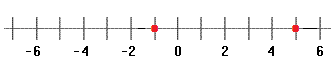A −1 − 5 B |−1 − 5| C −1 + 5 D |−1 + 5|
Question 12 Explanation:
Remember that distance is always a positive value, regardless of the signs of the points involved. So, the correct answer must include an absolute value. After that, all that is necessary is to accurately label the points.

The first point must be −1, as it is midway between −2 and 0. The second point must be 5 because it is halfway between 4 and 6. Subtract one point from the other and place the expression inside of an absolute value to find the correct answer:

AB = |ba| or |ab|
= |−1 − 5|
 Question 13
8.32 × 3.2 =

 A 26.624 B 266.24 C 41.6 D 0.416
Question 13 Explanation:
In order to multiply decimal values together, right align the values vertically and multiply as usual, ignoring the decimal points until the product is found: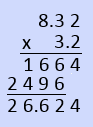Placement of the decimal point in the product is determined by the number of digits to the right of the decimal points in the values multiplied. In this case, there are three digits to the right of the decimal points: 32 and 2, so the product must also have three digits to the right of the decimal: 26624 becomes 26.624 to satisfy this condition.
 Question 14
Arrange the following fractions in order from least to greatest.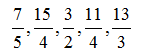AB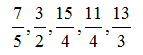C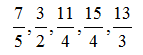D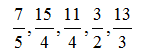Question 14 Explanation:
There are two useful methods for directly comparing the values of fractions: converting each fraction to a decimal and rewriting each fraction with a common denominator. In this case, the least common denominator would be 60 (5 * 4 * 3), and as a result, the optimal approach entails converting each fraction to a decimal, instead:Now, order the decimals from least to greatest, and match the resulting list with its corresponding list of fractions: 1.4, 1.5, 2.75, 3.75, and 4.33 which corresponds to: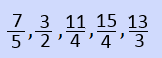Once you are finished, click the button below. Any items you have not completed will be marked incorrect.
There are 14 questions to complete.
 ← List →